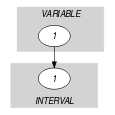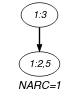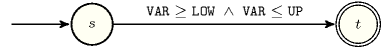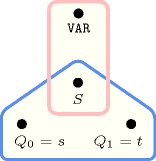## 5.178. in_interval

Origin

Domain definition.

Constraint

$\mathrm{𝚒𝚗}_\mathrm{𝚒𝚗𝚝𝚎𝚛𝚟𝚊𝚕}\left(\mathrm{𝚅𝙰𝚁},\mathrm{𝙻𝙾𝚆},\mathrm{𝚄𝙿}\right)$

Synonyms

$\mathrm{𝚍𝚘𝚖}$, $\mathrm{𝚒𝚗}$.

Arguments
 $\mathrm{𝚅𝙰𝚁}$ $\mathrm{𝚍𝚟𝚊𝚛}$ $\mathrm{𝙻𝙾𝚆}$ $\mathrm{𝚒𝚗𝚝}$ $\mathrm{𝚄𝙿}$ $\mathrm{𝚒𝚗𝚝}$
Restriction
$\mathrm{𝙻𝙾𝚆}\le \mathrm{𝚄𝙿}$
Purpose

Enforce the domain variable $\mathrm{𝚅𝙰𝚁}$ to take a value within the interval $\left[\mathrm{𝙻𝙾𝚆},\mathrm{𝚄𝙿}\right]$.

Example
$\left(3,2,5\right)$

The $\mathrm{𝚒𝚗}_\mathrm{𝚒𝚗𝚝𝚎𝚛𝚟𝚊𝚕}$ constraint holds since its first argument $\mathrm{𝚅𝙰𝚁}=3$ is greater than or equal to its second argument $\mathrm{𝙻𝙾𝚆}=2$ and less than or equal to its third argument $\mathrm{𝚄𝙿}=5$.

Typical
 $\mathrm{𝙻𝙾𝚆}<\mathrm{𝚄𝙿}$ $\mathrm{𝚅𝙰𝚁}>\mathrm{𝙻𝙾𝚆}$ $\mathrm{𝚅𝙰𝚁}<\mathrm{𝚄𝙿}$
Symmetries
• $\mathrm{𝙻𝙾𝚆}$ can be decreased.

• $\mathrm{𝚄𝙿}$ can be increased.

• An occurrence of a value of $\mathrm{𝚅𝙰𝚁}$ can be replaced by any other value in $\left[\mathrm{𝙻𝙾𝚆},\mathrm{𝚄𝙿}\right]$.

• One and the same constant can be added to $\mathrm{𝚅𝙰𝚁}$, $\mathrm{𝙻𝙾𝚆}$ and $\mathrm{𝚄𝙿}$.

Remark

Entailment occurs immediately after posting this constraint.

The $\mathrm{𝚒𝚗}_\mathrm{𝚒𝚗𝚝𝚎𝚛𝚟𝚊𝚕}$ constraint is referenced under the name $\mathrm{𝚍𝚘𝚖}$ in

Systems

member in Choco, dom in Gecode, in in JaCoP, in in SICStus.

generalisation: $\mathrm{𝚒𝚗}_\mathrm{𝚒𝚗𝚝𝚎𝚛𝚟𝚊𝚕}_\mathrm{𝚛𝚎𝚒𝚏𝚒𝚎𝚍}$ (reified version), $\mathrm{𝚒𝚗}_\mathrm{𝚒𝚗𝚝𝚎𝚛𝚟𝚊𝚕𝚜}$ (single interval replaced by a set of intervals), $\mathrm{𝚒𝚗}_\mathrm{𝚜𝚎𝚝}$ (interval replaced by set variable).

Keywords
Derived Collections
 $\mathrm{𝚌𝚘𝚕}\left(\mathrm{𝚅𝙰𝚁𝙸𝙰𝙱𝙻𝙴}-\mathrm{𝚌𝚘𝚕𝚕𝚎𝚌𝚝𝚒𝚘𝚗}\left(\mathrm{𝚟𝚊𝚛}-\mathrm{𝚍𝚟𝚊𝚛}\right),\left[\mathrm{𝚒𝚝𝚎𝚖}\left(\mathrm{𝚟𝚊𝚛}-\mathrm{𝚅𝙰𝚁}\right)\right]\right)$ $\mathrm{𝚌𝚘𝚕}\left(\begin{array}{c}\mathrm{𝙸𝙽𝚃𝙴𝚁𝚅𝙰𝙻}-\mathrm{𝚌𝚘𝚕𝚕𝚎𝚌𝚝𝚒𝚘𝚗}\left(\mathrm{𝚕𝚘𝚠}-\mathrm{𝚒𝚗𝚝},\mathrm{𝚞𝚙}-\mathrm{𝚒𝚗𝚝}\right),\hfill \\ \mathrm{𝚒𝚝𝚎𝚖}\left(\mathrm{𝚕𝚘𝚠}-\mathrm{𝙻𝙾𝚆},\mathrm{𝚞𝚙}-\mathrm{𝚄𝙿}\right)\right]\hfill \end{array}\right)$
Arc input(s)

$\mathrm{𝚅𝙰𝚁𝙸𝙰𝙱𝙻𝙴}$ $\mathrm{𝙸𝙽𝚃𝙴𝚁𝚅𝙰𝙻}$

Arc generator
$\mathrm{𝑃𝑅𝑂𝐷𝑈𝐶𝑇}$$↦\mathrm{𝚌𝚘𝚕𝚕𝚎𝚌𝚝𝚒𝚘𝚗}\left(\mathrm{𝚟𝚊𝚛𝚒𝚊𝚋𝚕𝚎},\mathrm{𝚒𝚗𝚝𝚎𝚛𝚟𝚊𝚕}\right)$

Arc arity
Arc constraint(s)
 $•\mathrm{𝚟𝚊𝚛𝚒𝚊𝚋𝚕𝚎}.\mathrm{𝚟𝚊𝚛}\ge \mathrm{𝚒𝚗𝚝𝚎𝚛𝚟𝚊𝚕}.\mathrm{𝚕𝚘𝚠}$ $•\mathrm{𝚟𝚊𝚛𝚒𝚊𝚋𝚕𝚎}.\mathrm{𝚟𝚊𝚛}\le \mathrm{𝚒𝚗𝚝𝚎𝚛𝚟𝚊𝚕}.\mathrm{𝚞𝚙}$
Graph property(ies)
$\mathrm{𝐍𝐀𝐑𝐂}$$=1$

Graph model

Parts (A) and (B) of Figure 5.178.1 respectively show the initial and final graph associated with the Example slot. Since we use the $\mathrm{𝐍𝐀𝐑𝐂}$ graph property, the unique arc of the final graph is stressed in bold.

##### Figure 5.178.1. Initial and final graph of the $\mathrm{𝚒𝚗}_\mathrm{𝚒𝚗𝚝𝚎𝚛𝚟𝚊𝚕}$ constraint(a) (b)
Automaton

Figure 5.178.2 depicts the automaton associated with the $\mathrm{𝚒𝚗}_\mathrm{𝚒𝚗𝚝𝚎𝚛𝚟𝚊𝚕}$ constraint. We have a single 0-1 signature variable $S$ as well as the following signature constraint: $\mathrm{𝚅𝙰𝚁}\ge \mathrm{𝙻𝙾𝚆}\wedge \mathrm{𝚅𝙰𝚁}\le \mathrm{𝚄𝙿}⇔S$.

##### Figure 5.178.2. Automaton of the $\mathrm{𝚒𝚗}_\mathrm{𝚒𝚗𝚝𝚎𝚛𝚟𝚊𝚕}$ constraint##### Figure 5.178.3. Hypergraph of the reformulation corresponding to the automaton of the $\mathrm{𝚒𝚗}_\mathrm{𝚒𝚗𝚝𝚎𝚛𝚟𝚊𝚕}$ constraint